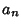## Boustrophedon Transform

The boustrophedon (ox-plowing'') transform b of a sequence a is given by(1)(2)

for, whereis a Secant Number or Tangent Number defined by(3)

The exponential generating functions of a and b are related by(4)

where the exponential generating function is defined by(5)

See also Alternating Permutation, Entringer Number, Secant Number, Seidel-Entringer-Arnold Triangle, Tangent Number

References

Millar, J.; Sloane, N. J. A.; and Young, N. E. A New Operation on Sequences: The Boustrophedon Transform.'' J. Combin. Th. Ser. A 76, 44-54, 1996.